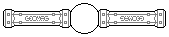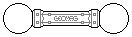Web textodigital.com
Construction of the reinforced rhombicosidodecahedron, method 1
It is possible to build a rhombicosidodecahedron with an internal structure which makes it much stronger. This reinforced solid allows for building certain models which would not be possible using the normal version. For instance, this model inspired in The Brussels' Atomium.
This structure was published in 2005 in the Geomag forum by a member nicknamed Chillum. Although the original message was lost, it has been reposted recently (as of January 2007), including pictures and a link to a VRML version of the object.
Familiarity with the normal rhombicosidodecahedron is required prior to trying to build the reinforced one. Build one once or twice and examine it in detail until you feel well acquainted with it.
The reinforced version uses pentagonal pyramids instead of pentagonal panels. Their bases are the pentagonal faces, and they are placed pointing inwards. So, we will not use any pentagon, but we'll need 5 × 12 = 60 additional rods and 12 balls for the pyramids.
Each pyramid apex will be joined by two rods to two vertices of an auxiliary cube placed at the geometric center of the object. This will require 12 rods, 6 squares and 8 balls for the cube, and 2 × 12 = 24 additional rods for the joins between the cube and the pyramids.
So, the object can be built with 80 balls, 216 rods, and 36 squares. A reinforced rhombicosidodecahedron will be about 60% heavier than a normal one, but, also, much stronger.
It can be shown, mathematically, that the auxiliary internal structure is exact, that is, it will fit exactly if the model is built with precise enough pieces (as Geomag pieces use to be).
It is not easy to write detailed and precise building instructions, and we will not even try. The following pictures, and the explanation above, should suffice.
We use red rods for the true edges, and silver rods for the auxiliary structure. This is the minimum structure required to put in place, and stabilize, the central cube: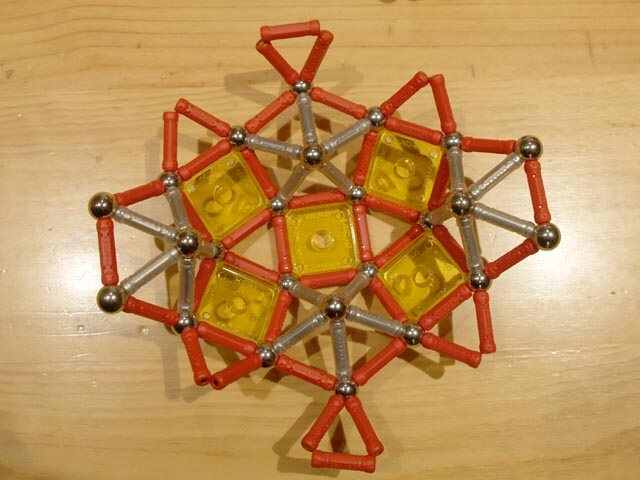Construction of the reinforced rhombicosidodecahedron, method 1, step 1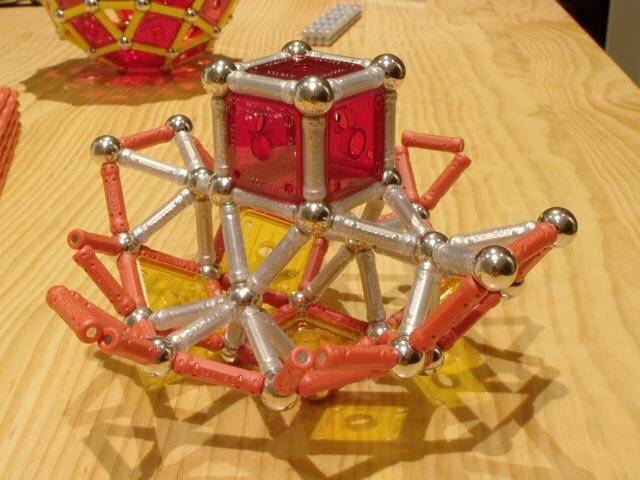Construction of the reinforced rhombicosidodecahedron, method 1, step 2
Two rear pentagonal pyramids have been added here. Two opposite pyramids will be built now, their apices being the two balls seen in the foreground: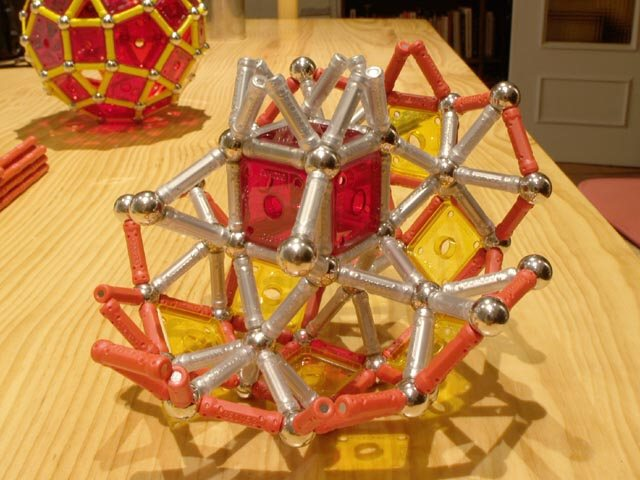Construction of the reinforced rhombicosidodecahedron, method 1, step 3
The pair of front pyramids has been built, and the object has rotated about 45 degrees clockwise around its vertical axis. The four upper balls are the apices of the four remaining pyramids.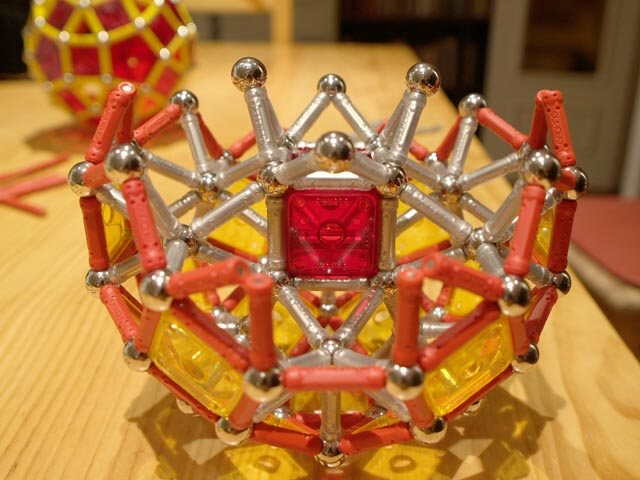Construction of the reinforced rhombicosidodecahedron, method 1, step 4
Front and rear pyramids added. Just two side pyramids remain: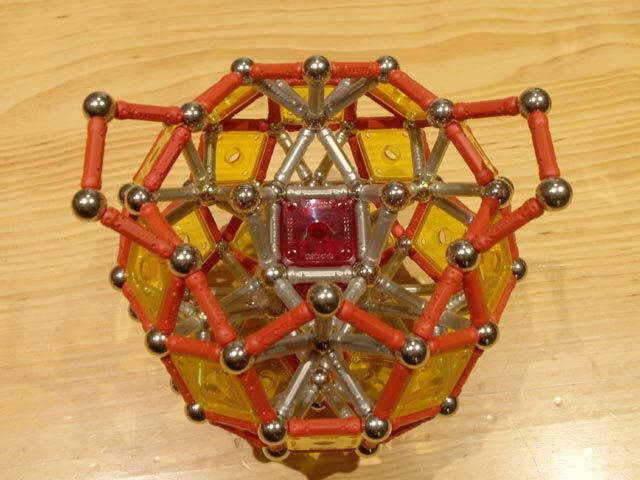Construction of the reinforced rhombicosidodecahedron, method 1, step 5
Done!: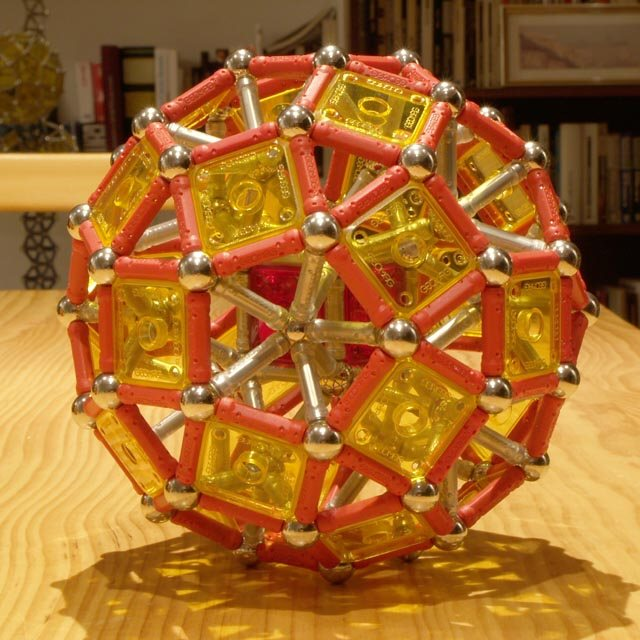Reinforced rhombicosidodecahedron, method 1 332 pieces: 80 balls, 216 rods, 36 squares (1.77 kg)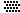Notice that six of the thirty square faces are parallel to those of the central cube. In fact, all thirty squares can be classified in five similar subsets, each one of them corresponding to a different possible orientation of the cube.